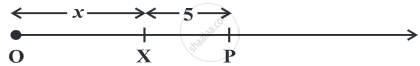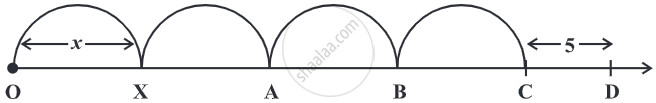# Algebraic Expressions

#### Topics

• Variable
• Constant
• Algebraic Expressions
• Value of Expression
• Number line and an expression

## Definition

• Variable: Variable means something that can vary, i.e. change.
• Constant: Constant term is a term in an algebraic expression that has a value that is constant or cannot change because it does not contain any modifiable variables.
• Algebraic expressions: Algebraic expressions are formed from variables and constants. We use the operations of addition, subtraction, multiplication, and division on the variables and constants to form expressions.

# Algebraic Expressions:

## 1. Variable:

• The word variable means something that can vary, i.e. change.

• A variable takes on different numerical values; its value is not fixed.

• Variables are denoted usually by letters of the alphabets, such as x, y, z, l, m, n, p, etc.

• From variables, we form expressions.

## 2. Constant:

• A constant term is a term in an algebraic expression that has a value that is constant or cannot change because it does not contain any modifiable variables.

• Example, x2 + 2x + 3, the 3 is a constant term.

## 3. Algebraic expressions:

• Algebraic expressions are formed from variables and constants. We use the operations of addition, subtraction, multiplication, and division on the variables and constants to form expressions.

• For example, the expression 4xy + 7 is formed from the variables x and y and constants 4 and 7. The constant 4 and the variables x and y are multiplied to give the product 4xy and the constant 7 is added to this product to give the expression.

## 4. Value of an expression:

• The expressions are formed by performing operations like addition, subtraction, multiplication, and division on the variables.

• From y, we formed the expression (10y – 20). For this, we multiplied y by 10 and then subtracted 20 from the product.

• The value of an expression thus formed depends upon the chosen value of the variable.
when y =15, 4 y + 5 = 4 × 15 + 5 = 65;
when y =0, 4 y + 5 = 4 × 0 + 5 = 5.

#### 6. Number line and an expression:

1) Consider the expression x + 5.Let us say the variable x has a position X on the number line; X may be anywhere on the number line, but it is definite that the value of x + 5 is given by a point P, 5 units to the right of X.

2) What about the position of 4x and 4x + 5?The position of 4x will be point C; the distance of C from the origin will be four times the distance of X from the origin. The position D of 4x + 5 will be 5 units to the right of C.

If you would like to contribute notes or other learning material, please submit them using the button below.

### Shaalaa.com

How are Algebraic Expressions Formed? [00:12:48]
S
0%